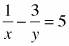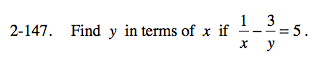### Home > PC > Chapter 2 > Lesson 2.3.6 > Problem2-147

2-147.

Find y in terms of x if. Homework Help ✎Multiply each and every term by xy. Simplify.

Get all of the y-terms on one side of the equation and everything without a y on the other side.

Factor out y.

Divide both sides by the expression (1 − 5x).

$xy\frac{1}{x}-xy\frac{3}y=5xy$

$y-3x=5xy$

$y-5xy=3x$

$y(1-5x)=3x$

$\frac{3x}{1-5x}$